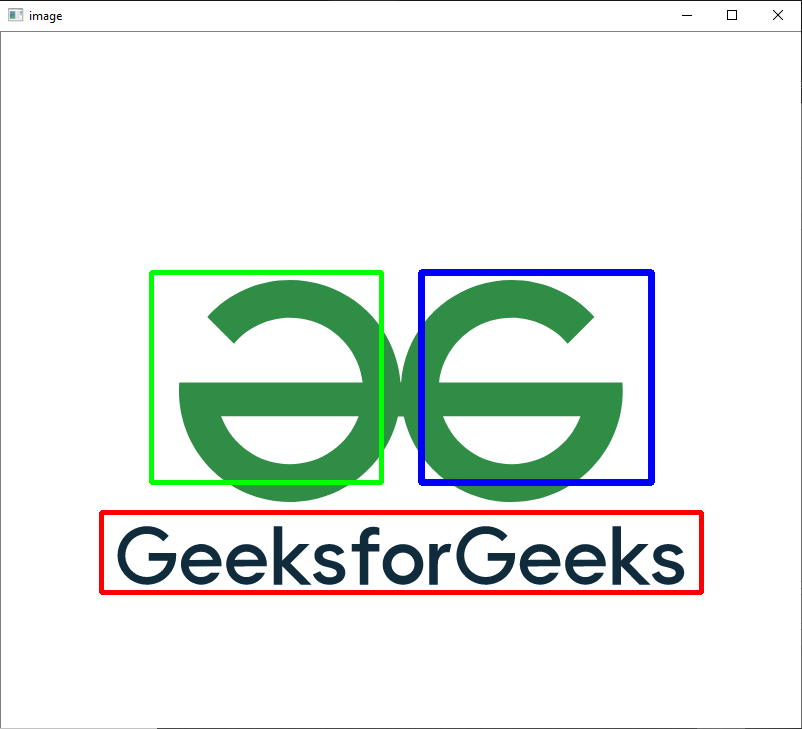# Draw Multiple Rectangles in Image using Python-Opencv

• Last Updated : 17 Oct, 2021

In this article, we are going to see how to draw multiple rectangles in an image using Python and OpenCV.

## Function used:

Attention geek! Strengthen your foundations with the Python Programming Foundation Course and learn the basics.

To begin with, your interview preparations Enhance your Data Structures concepts with the Python DS Course. And to begin with your Machine Learning Journey, join the Machine Learning - Basic Level Course

• rectangle(): In the OpenCV, the cv2.rectangle function is used to draw a rectangle on the image in Python.

Syntax: cv2.rectangle(image, starting_coordinate, ending_coordinate, color, thickness)

• imshow(): In the OpenCV, the cv2.imshow() function is used to display an image in Python.

Syntax: cv2.imshow(window_name, image)

• waitKey(): In the OpenCV, the cv2.waitkey() function allows you to wait for a specific time in milliseconds.
• destroyAllWindows(): In the OpenCV, the destroyAllWindows() function is used to closes all the windows created using the OpenCV methods.

## Python3

 `# importing OpenCV(cv2) module``import` `cv2`` ` `# Read RGB image``img ``=` `cv2.imread(``"D:\Naveen\gfg.PNG"``)`` ` `# Draw rectangles``# Red rectangle``cv2.rectangle(img, (``100``, ``560``), (``700``, ``480``),``              ``(``0``, ``0``, ``255``), ``3``)`` ` `# Blue rectangle``cv2.rectangle(img, (``650``, ``450``), (``420``, ``240``),``              ``(``255``, ``0``, ``0``), ``5``)`` ` `# Green rectangle``cv2.rectangle(img, (``150``, ``450``), (``380``, ``240``),``              ``(``0``, ``255``, ``0``), ``4``)`` ` `# Output img with window name as 'image'``cv2.imshow(``'image'``, img)`` ` `# Maintain output window utill``# user presses a key``cv2.waitKey(``0``)`` ` `# Destroying present windows on screen``cv2.destroyAllWindows()`

Output:My Personal Notes arrow_drop_up